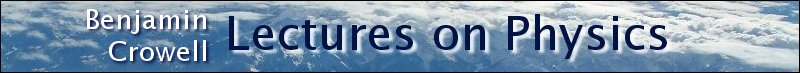Lectures on Physics has been derived from Benjamin Crowell's Light and Matter series of free introductory textbooks on physics. See the editorial for more information....Question: The speed of light is 3.0x108 m/s. What is the wavelength of the radio waves emitted by KLON, a station whose frequency is 88.1 MHz?

Solution: Solving for wavelength, we have

λ = v/f

= (3.0x108 m/s)/(88.1x106 s-1)

= 3.4 m

The size of a radio antenna is closely related to the wavelength of the waves it is intended to receive. The match need not be exact (since after all one antenna can receive more than one wavelength!), but the ordinary "whip" antenna such as a car's is 1/4 of a wavelength. An antenna optimized to receive KLON's signal (which is the only one my car radio is ever tuned to) would have a length of 3.4 m/4 = 0.85 m.

Last Update: 2010-11-11